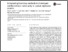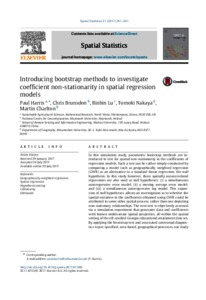# Introducing bootstrap methods to investigate coefficient non-stationarity in spatial regression models

Harris, Paul and Brunsdon, Chris and Lu, Binbin and Nakaya, Tomoki and Charlton, Martin (2017) Introducing bootstrap methods to investigate coefficient non-stationarity in spatial regression models. Spatial Statistics, 21 (1). pp. 241-261. ISSN 2211-6753Previewmore...Add this article to your Mendeley library

## Abstract

In this simulation study, parametric bootstrap methods are introduced to test for spatial non-stationarity in the coefficients of regression models. Such a test can be rather simply conducted by comparing a model such as geographically weighted regression(GWR) as an alternative to a standard linear regression, the null hypothesis. In this study however, three spatially autocorrelated regressions are also used as null hypotheses: (i) a simultaneous autoregressive error model; (ii) a moving average error model; and (iii) a simultaneous autoregressive lag model. This expansion of null hypotheses, allows an investigation as to whether the spatial variation in the coefficients obtained using GWR could be attributed to some other spatial process, rather than one depicting non-stationary relationships. The new test is objectively assessed via a simulation experiment that generates data and coefficients with known multivariate spatial properties, all within the spatial setting of the oft-studied Georgia educational attainment data set. By applying the bootstrap test and associated contextual diagnostics to pre-specified, area-based, geographical processes, our studyprovides a valuable steer to choosing a suitable regression model for a given spatial process.

Item Type: Article Geographically weighted regression; Spatial regression; Hypothesis testing; Collinearity; GWmodel Faculty of Social Sciences > Geography 10977 https://doi.org/10.1016/j.spasta.2017.07.006 Martin Charlton 07 Aug 2019 14:51 Spatial Statistics Elsevier YesItem control page